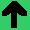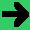# The Basic Idea of Finite State Automata

• The basic idea is that there are a set of states.
• At any time, the system is in exactly one state (it's discrete).
• When the system starts, it is in a particular state.
• There are also a set of possible inputs.
• The system reads the inputs one at a time.
• Each state has a list of transitions.
• For each input on each state there is a transition to a state.
• The input is consumed.
• There are some other decorations and formalities, but that is roughly it.